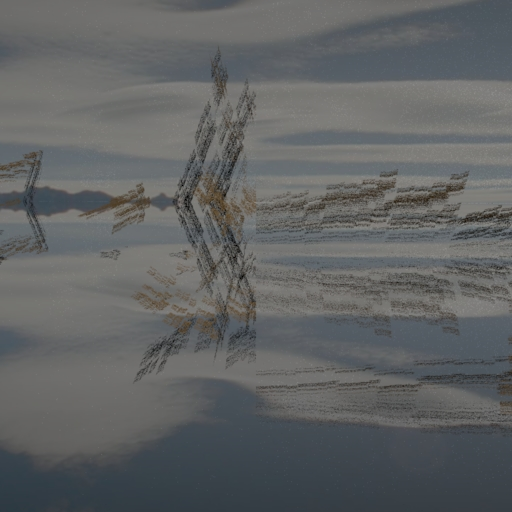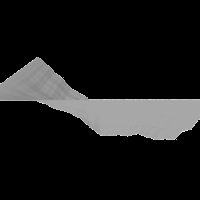Rendering Fractal Interpolation Surfaces

## Abstract

Given a height field, I would like to represent it as the graph of a fractal interpolation function h(x, y) over a compact domain D in R2. Fractal interpolation functions (FIFs) are always continuous, but can be smooth or roughdepending on the choice of shear factors. FIFs can interpolate any number of points in R3. There exist algorithms for ray tracing and rendering fractals which work for FIFs. There is also a slightly less known advantage of FIFs: we can integrate over them using a relatively small number of additions and multiplications. In this project I investigated and implemented a rendering algorithm for a special type of FIF, namely tensor product fractal surfaces. Although I did not get to fully exploit the advantages of the efficient integrability of FIFs in this project, there appears to be worthwhile future work ahead in which I can explore these ideas.

## Introdution

Fractals are commonly used in graphics and have the advantage of being arbitrarily complex, yet are relatively easy to implement. A special class of fractals are those generated by iteratively applying contractive affine transformations to any compact set. The iterative process converges quickly to a fractal. Such fractals are termed self-affine since a part of the fractal can be obtained by simply applying an affine transformation to the whole fractal. The set of transformations used to generate a self-affine fractal is known as an iterated function system, abbreviated IFS. For more on IFS and self-affine fractals, see Michael Barnsley's excellent book on the subject, Fractals Everywhere1.

Among the many types of fractals that IFS can create are fractal interpolation functions. Given N + 1 points

(x0, y0), ..., (xN, yN)

we can construct a fractal function interpolating those points, as described in Chapter VI of Barnsley's book. These functions have many interesting properties, including the fact that we can compute the integral of such a function over the interval [x0, xN]. Not only can we compute the integral of a fractal interpolation function f(x), we can also integrate xf(x), f 2(x), and more.

In computer graphics, we are interested in rendering images of complex objects using techniques such as ray tracing. In ray tracing, we shoot rays in various directions throughout the scene to eventually determine how much light is hitting or reflected from a given point in a scene, until we can finally assign a single color to every pixel in our viewing window. One useful way to model objects for ray tracing is a microfacet model, where every object is represented as a set of small planar reflectors, so whenever light hits a particular one of those mirrors, we can determine how it is reflected out of the object at that point simply based on the normal vector of that mirror.

In the real world, objects appear to be not just microfacets, but rather bumpy at various scales. For instance, a rock as seen by the human eye has a certain arrangement of microfacets on its surface, but at a smaller scale it may have more complex microfacets within a single microfacet, and so forth into smaller and smaller scales.

Fractals exhibit a similar type of complexity across various scales. This makes them computationally easy to manage in certain ways. Since many real world objects exhibit types of self-similarity across scales, it is an interesting possibility that fractal representations of certain objects can be useful in practical applications.

In this project I focused on implementing a rendering algorithm for 2D tensor product fractal interpolation functions. Such functions are constructed as follows. Given two arbitrary fractal interpolation functions f(x) over the interval [x0, xN] and g(y) over the interval [y0, yM], we can define their tensor product fractal function to be h(x, y) = f(x)g(y) over the domain [x0, xN] x [y0, yM]. Following the work in a paper2 I came across on this topic, I found a general method for rendering such surfaces, as well as some theory on how integrability of these functions helps in the rendering process.

The basic difficulty is simple to state: if we are given a description of a fractal surface, which could potentially be infinitely bumpy and rough, how on earth would we calculate normal vectors over it in order to do ray tracing? The answer rests in the following key observation: if we define a function F(x) to be the integral of a fractal interpolation function f(x) over an interval [x0, x], then F(x) is itself a fractal interpolation function. But, since F(x) is the integral of a continuous function, we can take its derivative. Hence, for a tensor product fractal function H(x,y) = F(x)G(y) where F(x) and G(y) are integrals of fractal interpolation functions f(x) and g(y), we can compute the derivatives Hx (x, y) = F'(x)G(y) = f(x)G(y) and Hy(x, y) = F(x)G' (y) = F(x)g(y) with respect to x and y respectively which gives us two tangent vectors on the graph of H(x, y) at (x, y), from which the cross product yields the normal vector N(x, y). The paper2 describes how these observations lead to a ray-intersection algorithm for tensor product FIF surfaces.

## Problem

### Input

The geometric description of a scene, consisting of objects modeled by tensor product fractal interpolation functions that are each a product of two 1D FIFs.

### Output

A ray-traced image of the scene, where each tensor product FIF surface is rendered using the ray-intersection algorithm for these surfaces.

## Results

The basic ray intersection algorithm I implemented appears to have worked for simple scenes, but I think there was still some bug either in how I constructed one of my scenes, or there is still a small bug somewhere in the rendering code itself. These are the pictures I showed at the rendering competition. For the color image I only showed a small version at the rendering competition as the big version was still being rendered. However the big version revealed that there is some problem with the code that I have not yet looked into.The color image consists of a simple texture map at the top of the image that looks like a sky with clouds, while below that area is a "sea" which is actually a shiny metal fractal interpolation surface. Although these surfaces tend to look smooth at a glance, the way light reflects off of these surfaces is sometimes rather peculiar and interesting, as things are in the real world. There is also another fractal interpolation surface which shows the bug I mentioned earlier that looks like the brown lines scattered throughout the image. Its image is also reflected off the surface of the fractal sea. A fractal sea may be an interesting way to display the irregularity of water in real life, unlike the rather regular spherical beads of water drops and perfect circularity of water waves that are often seen in synthesized images. I am not too familiar with work that has been done in this area, however.

Of course matching the real world phenomena using mathematical and physical descriptions is the central problem in realistic image synthesis, and it is still not clear exactly how fractals can be useful in reaching these goals. However I think it is useful to explore some of the advantages of certain types of fractals that possess computational advantages that may make them more useful in modeling and rendering.

## References

1 M. Barnsley. Fractals Everywhere, 2nd ed., Academic Press, 1993.
2 W. Cochran, R. Lewis, J. Hart. The Normal of a Fractal Surface. The Visual Computer 17 (4): 209-218, 2001.